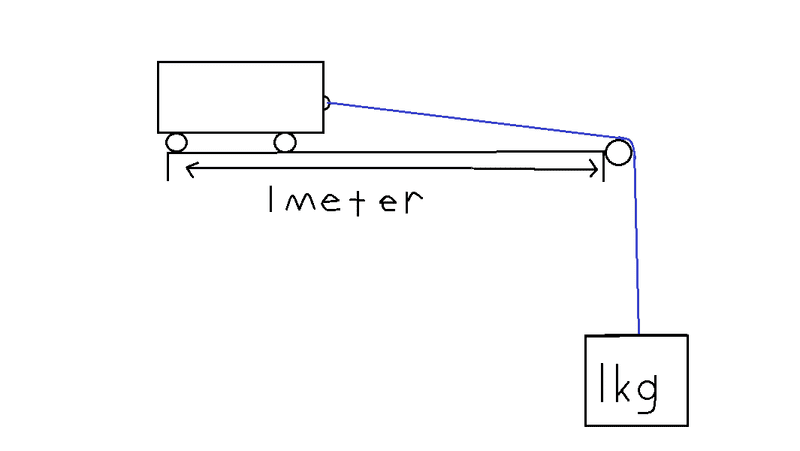# Basic calculate time question

hunter45
I have this question from another site where a member asked this. I attempted to answer it and they think it is incorrect. I would therefore like someone who knows what they are talking about to answer this question if it is incorrect and explain where I am going wrong. Thank you.Question : How long will it take to travel that 1 metre?
NOTE: The surface is a frictionless surface. The mass of the trolley is 0.5kg

My method:

F = ma , F = (0.5+1) X 9.8 = 14.7N
W= Fd , W = 14.7 X 1 = 14.7J

Ek = 1/2mv^2
14.7 = 0.5 X 1.5 X v^2
v^2 = 19.6, v = 4.427 m/s

v = d/t
t = d/s
t = 1/4.427
t = 0.226s

mikeph
In the first step you're incorrect to say the force is 14.7 N because only the weight of the 1kg block is acting to accelerate the apparatus. The weight of the 0.5 kg block is being cancelled by the reaction force of the table. In essence you have a 1.5 kg block being accelerated by a 9.8 N force.

In your next step you're correct to equate work done with final kinetic energy, but incorrect to equate the final velocity with the average velocity of the apparatus, since the apparatus is accelerating.

Energy balance is one way to do this but a faster way is to stick with the equations of motion. The acceleration is F/m = 9.8/1.5 = 6.53 m/s^2, the starting velocity is 0 m/s and the distance travelled is 1 m, so your time answer is found using:

s = 0.5*a*t^2 + v*t

this gives you t^2 = 1/(0.5*6.53); t = 0.55 s.

hunter45
In the first step you're incorrect to say the force is 14.7 N because only the weight of the 1kg block is acting to accelerate the apparatus. The weight of the 0.5 kg block is being cancelled by the reaction force of the table. In essence you have a 1.5 kg block being accelerated by a 9.8 N force.

In your next step you're correct to equate work done with final kinetic energy, but incorrect to equate the final velocity with the average velocity of the apparatus, since the apparatus is accelerating.

Energy balance is one way to do this but a faster way is to stick with the equations of motion. The acceleration is F/m = 9.8/1.5 = 6.53 m/s^2, the starting velocity is 0 m/s and the distance travelled is 1 m, so your time answer is found using:

s = 0.5*a*t^2 + v*t

this gives you t^2 = 1/(0.5*6.53); t = 0.55 s.
Thank you for a detailed, explained and very easy to understand explaination. I understand where I went wrong and why.

EDIT: If i was to use energy to calculate this problem, how would I do it?

Last edited:
mikeph
No problem!

Using energy, the work done in moving the apparatus 1 m under a force of 9.8 N is 9.8 J, which equates to a final velocity of 3.61 m/s. Since the acceleration is constant, the average velocity is equal to half the sum of the initial and final velocities, (0 + 3.61)/2 = 1.81 m/s. Then the time taken is just 1/1.81 = 0.55 s.

The two blocks can be treated together only because the chord keeps their velocities equal and their displacements are always in the same direction as the net force acting on them.

hunter45
No problem!

Using energy, the work done in moving the apparatus 1 m under a force of 9.8 N is 9.8 J, which equates to a final velocity of 3.61 m/s. Since the acceleration is constant, the average velocity is equal to half the sum of the initial and final velocities, (0 + 3.61)/2 = 1.81 m/s. Then the time taken is just 1/1.81 = 0.55 s.

The two blocks can be treated together only because the chord keeps their velocities equal and their displacements are always in the same direction as the net force acting on them.
Once again, you have provided a very well explained answer. Thank you Sir.